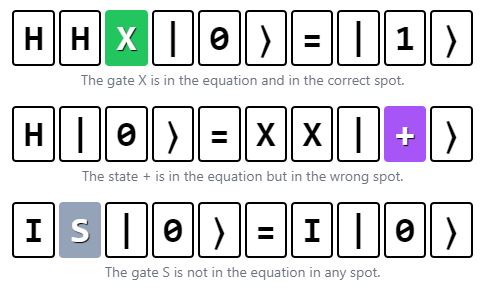Rating:
Quantle
5

# Quantle

Challenge your ability to solve quantum math equations with the puzzle method in Quantle. You will have hours of incredibly enjoyable gaming thanks to the gameplay's inspiration from the well-known Wordle puzzle game. Use your logical reasoning ability to make accurate predictions. Enjoy!

## How to play

The object of the game is to use the provided hints and the method of elimination to decipher the proper quantum computing equation within six tries. It is the qubit's state that is manipulated by quantum gates in each guess. The notation used to describe gates in mathematics There must be a "ket" on both sides of every correct equation. In quantum physics, the symbol "ket" is employed to denote particular quantum states. After making a guess, the cells' colors will update to reflect how close you came to the correct equation.The difficulty lies in determining which combination of gates and quantum states will produce the correct equation. To solve the puzzle, the player must utilize their knowledge of gates and quantum states to make educated estimates and fill in the puzzle pieces.

If you are a lover of numbers and the logic of mathematical equations, you can try Primle. Make predictions about 5-digit numbers as requested.

PUZZLE WORDLEVERSE NOT WORDS brain logic guess math quantle quantum
Comment (0)Be the first to comment